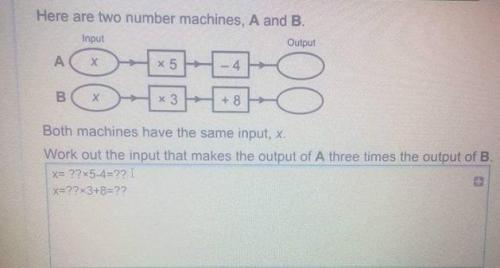# Solving Question - Linear Algebra (I believe)

## Recommended Posts

Hello all!

It's been quite a while since I posted here. I noticed this question while trying to help a niece solve a piece of homework and I just couldn't figure this one out. This isn't homework for me, trust me, I have left school long ago.

Could someone enlighten me as to the solution for this one and how to go about solving them? Just in case I need to assist with homework again in the future... at least then I'll be able to provide pointers. I also hate not being able to answer such things.

I had initially attempted to treat it as a simultaneous equation but I'm not convinced that it's the correct way to handle this one.

Thanks!Edited by RyanJ
##### Share on other sites

Ok, so you have A) y = 5x-4 and B) y = 3x+8. You want A = 3B, so

5x-4 = 3*(3x+8)

5x-4 = 9x + 24

4x = -28

x = -7

It's just a single equation since you only have one unknown.

It's presented as "two number machines," but the 3x condition links them together so you wind up with just the one equation.

Edited by KipIngram
##### Share on other sites

So, I was correct in my initial assumptions then? Ha, the irony.

I had attempted to solve it as a simultaneous equation but I figured that was the wrong method and abandoned it.

##### Share on other sites

Well, you could set it up as simultaneous equations by introducing a new variable:

3x+8 = y

5x-4 = 3y

which become

3x - y = -8

5x - 3y = 4

But that really makes the problem more complicated than it needs to be. Step one in solving that is to multiply the first equation by 3 and subtract them, which eliminates y.

## Create an account

Register a new account